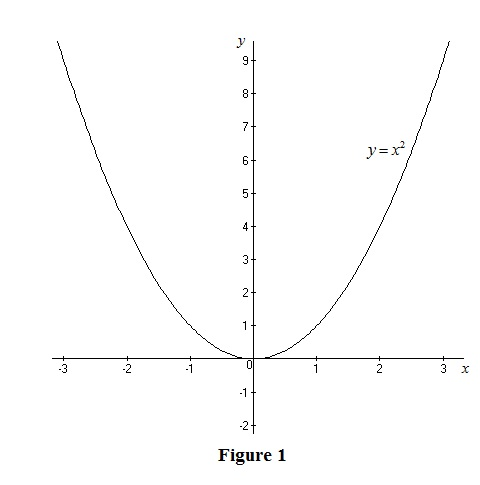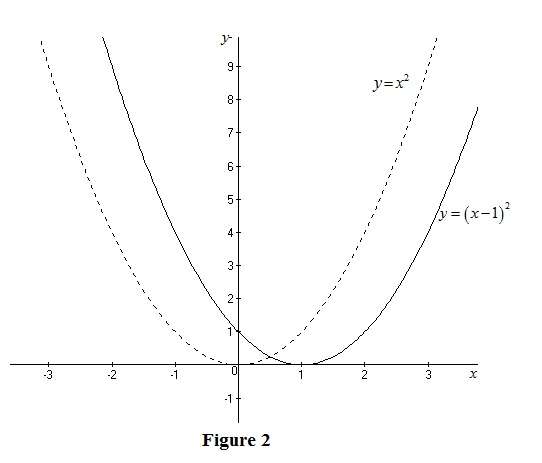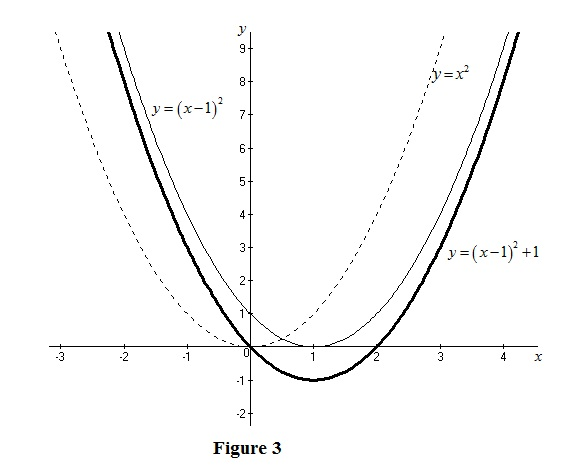# The graph of the function y = x 2 − 2 x + 2 by using transformation.### Single Variable Calculus: Concepts...

4th Edition
James Stewart
Publisher: Cengage Learning
ISBN: 9781337687805### Single Variable Calculus: Concepts...

4th Edition
James Stewart
Publisher: Cengage Learning
ISBN: 9781337687805

#### Solutions

Chapter 1, Problem 13RE
To determine

## To sketch: The graph of the function y=x2−2x+2 by using transformation.

Expert Solution

### Explanation of Solution

Given:

The function is, y=x22x+2.

Graph:

Express the given function as follows.

y=x22x+2=x22x+1+1=(x1)2+1

Thus, draw the graph of y=(x1)2+1.

The standard graph of the function y=x2 is roughly drawn as shown below in Figure 1.Then, draw the graph of y=(x1)2, shift the graph y=x2 by 1 unit to the right. Thus, the graph of y=(x1)2 is shown below in Figure 2.From Figure 2, it is observed that Figure 1 is shifted 1 unit to the right.

To draw the graph of y=(x1)2+1, shift the graph y=(x1)2 by 1 unit upward. Thus, the graph of y=(x1)2+1 is shown below in Figure 3.Observe that Figure 3 is obtained from Figure 1 in such a way that it is shifted 1 unit to the right and then shifted 1 unit upward.

### Have a homework question?

Subscribe to bartleby learn! Ask subject matter experts 30 homework questions each month. Plus, you’ll have access to millions of step-by-step textbook answers!